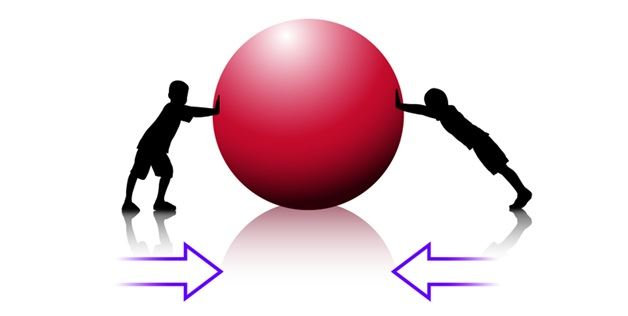# Types Of Forces## What is a Force?

Force is pushing or pulling any object resulting from the object’s interaction with another object. Without force, nothing can be moved, stopped or change the direction. It is a quantitative interaction between two physical bodies, between an object and its environment. There are various types of forces in nature.If an object is in its motion state than its current state will be either static or motion. Its position will only be changed if it is pushed or pulled. The external push or pull applied on the object changes its current state of motion is known as Force. This force can be of different types. They are stated below.

### Different Types Of Forces:

Force is a physical cause that can change the state of motion of any object or the dimensions. Different types of Forces are listed below-

#### Balanced Force:

When two forces are in opposite direction and possess equal magnitude then these forces called are a balanced force. Equal and opposite forces together acting on an object when it’s not in a motion is said to be in equilibrium.

#### Unbalanced Force:

The unbalanced force can be defined as ” when two forces acting on a body are of unequal size.

#### Frictional Force:

When you hit a ball and the ball is set into a motion, it stops after travelling a certain distance. Why? According to Newton’s first law, An object continues to be at rest or to be in a motion unless it is acted upon an external force. This external force is known as frictional force.

#### Contact Force:

Contact force is a force that acts as the point of contact between two objects.

• Spring Force: Spring is a tool used daily by many of us and their inertia are frequently neglected by assuming it as massless. It’s an extremely casual activity that a Spring, when strained, undergoes displacement when it is compacted it gets compressed and when its free it comes to its equilibrium position. This fact tells us that spring exerts an equal as well as an opposite force on a body which compresses or stretches it.

#### Non-contact Force:

The forces that act on bodies without being physically touched, are said to be non-contact forces. The forces that fall under the category of non-contact forces are stated below:

• Gravitational Force: Gravitation is a process by which bodies having physical weight gets attracted towards the earth surface. The force through a body attracts is proportional to their weight. Gravity helps in keeping all the planets in their respective orbit.
$\large F=\frac{Gm_{1}m_{2}}{r^{2}}$

Where,

F is force of gravitation in Newton

G is gravitational constant with value 6.673 ×× 10-11m3 kg-1s-2

m1 is mass of object 1

m2 is  mass of object 2

• Electrostatic Force: Electrostatics is defined as the attraction and repulsion of charges and is also known as other types of non-contact force. There are some materials that have special properties of producing charged on their surface. These then attract to opposite charges and hence shows the property of electrostatics.
• Magnetic Force:The magnetic force is a property by virtue of which some special substances, known as magnets exert a special force on substances that are made up of iron. This special force is called magnetic force.